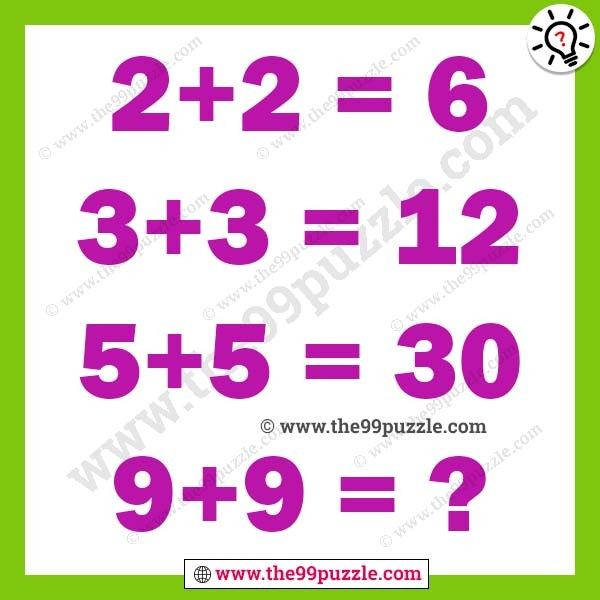# Tricky math equation with answer – Puzz152

Can you solve these brain teaser math puzzles? Get tricky math equation with answer. This type of math puzzles can improve your problem-solving skills. Can you solve the tricky math equation and calculate the last equation answer?

2+2=6

3+3=12

5+5=30

9+9=?2+2=2×2+2=6

3+3=3×3+3=12

5+5=5×5+5=30

9+9=9×9+9=90